Next: Optimization Up: Linear algebra Previous: Characteristic polynomial   Contents

Safe calculation of the spectral radius of a square real or interval matrix may be obtained with the procedures

int Spectral_Radius(INTERVAL_MATRIX &AA,double eps,double *ro,int iter)
int Spectral_Radius(MATRIX &AA,double eps,double *ro,int inter)
where
• AA: the matrix
• eps: a real that will be used to increment the solutions found for the median polynomial (i.e. the polynomial whose coefficients are the mid-point of the interval coefficients of the characteristic polynomial) until the polynomial evaluation does not contain 0
• ro: the upper bound of the spectral radius
• iter: the maximal number of allowed iteration
These procedures return 1 on success, 0 on failure (in which case increasing eps may be a good option) and -1 if the matrix is not square. The procedures

may also be used with a maximum number of iteration fixed to 100.

If it intended just to show that the spectral radius is larger than a given value seuil then you may use

int Spectral_Radius(INTERVAL_MATRIX &A,double eps,double *ro,double seuil);
int Spectral_Radius(MATRIX &A,double eps,double *ro,double seuil);
int Spectral_Radius(INTERVAL_MATRIX &A,double eps,double *ro,int iter,double seuil);
int Spectral_Radius(MATRIX &A,double eps,double *ro,int iter,double seuil);
Note that the calculation of the spectral radius may be used to check the regularity of an interval matrix. Indeed let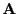be an interval matrix of dimension,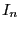the identity matrix of dimension. If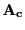is the mid-matrix ofwe may write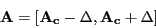Let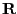be an arbitrary matrix. It can be shown that if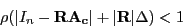where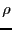denotes the spectral radius, thenis regular : this is known as the Beeck-Ris test.Next: Optimization Up: Linear algebra Previous: Characteristic polynomial   Contents
Jean-Pierre Merlet 2012-12-20